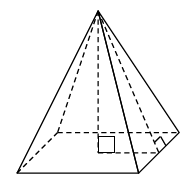### Home > INT2 > Chapter 11 > Lesson 11.2.1 > Problem11-53

11-53.What are the volume and surface area of a square-based right pyramid if the base edge has length $6$ units and the height of the pyramid is $4$ units?

$V=\frac{1}{3}(\text{base area})(\text{height})$

SA = total area of the $4$ faces and the base

Use the Pythagorean Theorem to find the height of the triangular faces.

$SA = 96\text{ u}^2$